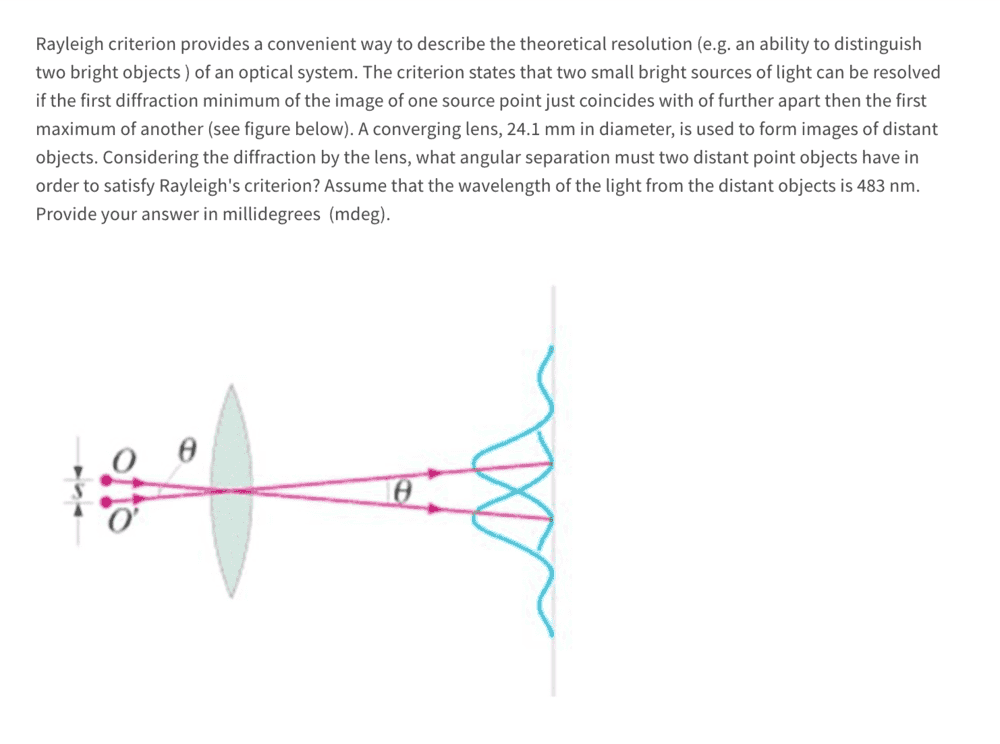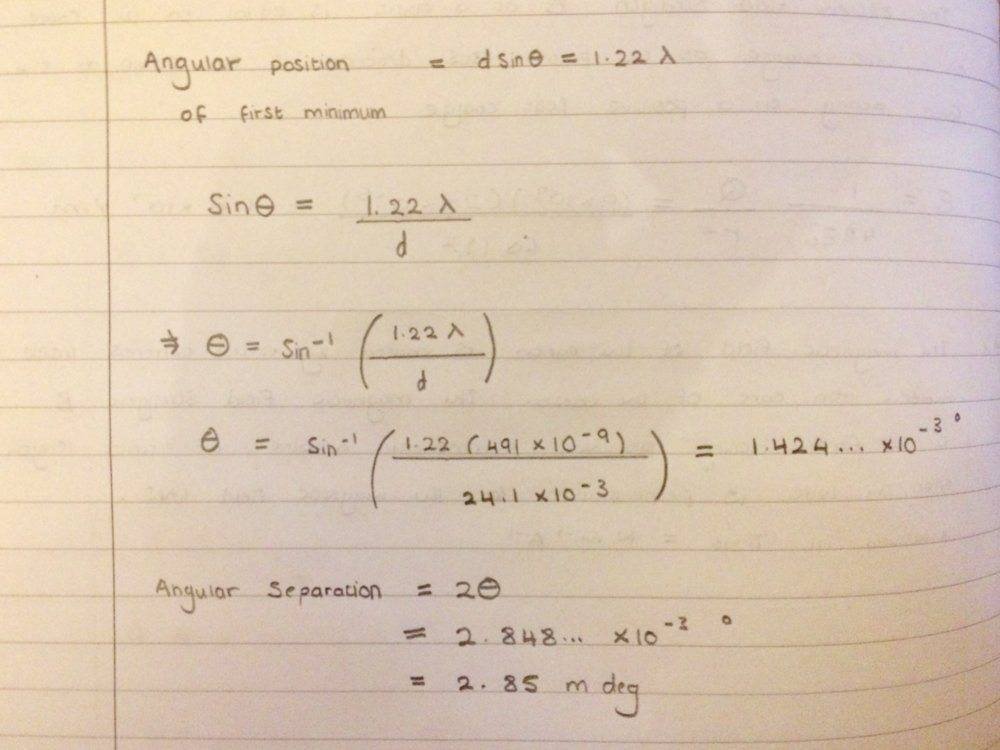# Rayleigh criterion: finding the angular separation between 2 distant points

Bolter
Homework Statement:
See below
Relevant Equations:
dsin(theta) = 1.22 lambdaI have tried this question and have gotten to an answer from the following stepsSo my angular separation is 2.85 millidegrees. Have I done this right with the formula I have made use of?

Any help would be great, thanks!

Cutter Ketch
All looks good until you get to the end and multiply the angle by two. There is no need to multiply by 2. Look at the diagram. Peak 1 is located at the first minimum of peak 2. That is a single multiple of the angle to the minimum. Yes, peak 2 is located at the first minimum of peak 1, but that is the same angle, not an additional angle.

P.S.: I like radians where for small angles sin(theta) ~= theta. Makes it easier to check the math, among other things.

•Bolter
Bolter
All looks good until you get to the end and multiply the angle by two. There is no need to multiply by 2. Look at the diagram. Peak 1 is located at the first minimum of peak 2. That is a single multiple of the angle to the minimum. Yes, peak 2 is located at the first minimum of peak 1, but that is the same angle, not an additional angle.

P.S.: I like radians where for small angles sin(theta) ~= theta. Makes it easier to check the math, among other things.

Yes understood now

Plus I've just realized I've been using the wrong wavelength. It should have been 483 nm NOT 491 nm. Making that adjustment I get the angle to be 1.4 millidegrees now